NEET  >  31 Year NEET Previous Year Questions: Alternating Current

# 31 Year NEET Previous Year Questions: Alternating Current

Test Description

## 35 Questions MCQ Test Physics Class 12 | 31 Year NEET Previous Year Questions: Alternating Current

31 Year NEET Previous Year Questions: Alternating Current for NEET 2023 is part of Physics Class 12 preparation. The 31 Year NEET Previous Year Questions: Alternating Current questions and answers have been prepared according to the NEET exam syllabus.The 31 Year NEET Previous Year Questions: Alternating Current MCQs are made for NEET 2023 Exam. Find important definitions, questions, notes, meanings, examples, exercises, MCQs and online tests for 31 Year NEET Previous Year Questions: Alternating Current below.
Solutions of 31 Year NEET Previous Year Questions: Alternating Current questions in English are available as part of our Physics Class 12 for NEET & 31 Year NEET Previous Year Questions: Alternating Current solutions in Hindi for Physics Class 12 course. Download more important topics, notes, lectures and mock test series for NEET Exam by signing up for free. Attempt 31 Year NEET Previous Year Questions: Alternating Current | 35 questions in 72 minutes | Mock test for NEET preparation | Free important questions MCQ to study Physics Class 12 for NEET Exam | Download free PDF with solutions
 1 Crore+ students have signed up on EduRev. Have you?
31 Year NEET Previous Year Questions: Alternating Current - Question 1

### Energy in a current carrying coil is stored in theform of   

Detailed Solution for 31 Year NEET Previous Year Questions: Alternating Current - Question 1

Energy stored in a coil =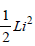where, L is the self-inductance and i the
current flowing through the inductor. Thus,
energy is stored in the magnetic field of the
coil.

31 Year NEET Previous Year Questions: Alternating Current - Question 2

### In a region of uniform magnetic inductionB = 10–2 tesla, a circular coil of radius 30 cm andresistance π2 ohm is rotated about an axis whichis perpendicular to the direction of B and whichforms a diameter of the coil. If the coil rotates at 200 rpm the amplitude of the alternating currentinduced in the coil is 

Detailed Solution for 31 Year NEET Previous Year Questions: Alternating Current - Question 2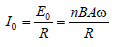Given, n = 1, B = 10–2 T,
A = π(0.3)2m2, R = π2
f= (200/60) and ω = 2π(200/60)
Substituting these values and solving, we
get
I0 = 6 × 10–3 A = 6mA

31 Year NEET Previous Year Questions: Alternating Current - Question 3

### In the circuit of Fig, the bulb will become suddenly bright if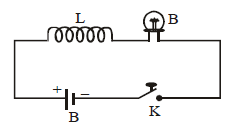Detailed Solution for 31 Year NEET Previous Year Questions: Alternating Current - Question 3

When contact is broken, current in the circuit decreases. To oppose the inductor releases current and emf is induced. This induced emf is having high value. Hence, The bulb will become suddenly bright.

The correct option is C.

31 Year NEET Previous Year Questions: Alternating Current - Question 4

In which of the following, emission of electronsdoes not take place 

Detailed Solution for 31 Year NEET Previous Year Questions: Alternating Current - Question 4

Thermionic emission : When a metal is
heated to a high temperature, the free
electrons gain kinetic energy and escape
from the surface of the metal.

Secondary emission : When an electron
strikes the surface of a metallic plate, it emits
other electrons from the surface.

Photoelectric emission : Emission of
electrons from the metal surface on irradiation

X-rays emission : High speed electrons
(emitted from a filament) striking a target
(a copper block) to emit X-rays.

31 Year NEET Previous Year Questions: Alternating Current - Question 5

The time constant of C–R circuit is 

Detailed Solution for 31 Year NEET Previous Year Questions: Alternating Current - Question 5

The time constant for resonance circuit,
C = CR
Growth of charge in a circuit containing
capacitance and resistance is given by the
formula,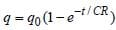CR is known as time constant in this formula.

31 Year NEET Previous Year Questions: Alternating Current - Question 6

In an a.c. circuit, the r.m.s. value of current, irms is related to the peak current, i0 by the relation

Detailed Solution for 31 Year NEET Previous Year Questions: Alternating Current - Question 6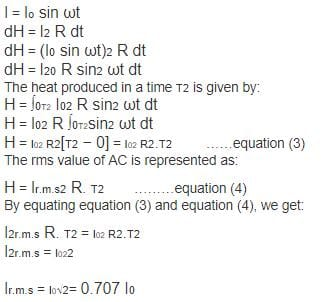31 Year NEET Previous Year Questions: Alternating Current - Question 7

An LCR series circuit is connected to a source of alternating current. At resonance, the applied voltage and the current flowing through the circuit will have a phase difference of 

Detailed Solution for 31 Year NEET Previous Year Questions: Alternating Current - Question 7

At resonance,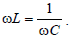The circuit
behaves as if it contains R only. So, phase
difference = 0
At resonance, impedance is minimum Zmin =
R and current is maximum, given by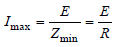It is interesting to note that before resonance
the current leads the applied emf, at
resonance it is in phase, and after resonance
it lags behind the emf. LCR series circuit is
also called as acceptor circuit and parallel
LCR circuit is called rejector circuit.

31 Year NEET Previous Year Questions: Alternating Current - Question 8

In an experiment, 200 V A.C. is applied at theends of an LCR circuit. The circuit consists ofan inductive reactance (XL ) = 50 Ω, capacitivereactance (XC ) = 50 Ω and ohmic resistance (R)= 10 Ω. The impedance of the circuit is

Detailed Solution for 31 Year NEET Previous Year Questions: Alternating Current - Question 8

Given : Supply voltage (Vac) = 200 V
Inductive reactance (XL) = 50 Ω
Capacitive reactance (XC) = 50 Ω
Ohmic resistance (R) = 10 Ω.
We know that impedance of the LCR circuit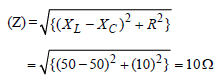31 Year NEET Previous Year Questions: Alternating Current - Question 9

In an A.C. circuit with voltage V and current I the power dissipated is 

Detailed Solution for 31 Year NEET Previous Year Questions: Alternating Current - Question 9

Power dissipated = Erms. Irms = (Erms) (Irms)
cos θ
Hence, power dissipated depends upon
phase difference.

31 Year NEET Previous Year Questions: Alternating Current - Question 10

The primary winding of a transformer has 500 turns whereas its secondary has 5000 turns. Theprimary is connected to an A.C. supply of 20 V,50 Hz. The secondary will have an output of        

Detailed Solution for 31 Year NEET Previous Year Questions: Alternating Current - Question 10

The transformer converts A.C. high voltage
into A.C. low voltage, but it does not cause
any change in frequency. The formula for
voltage is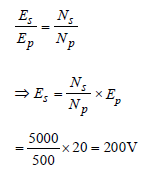Thus, output has voltage 200 V and
frequency 50 Hz.

31 Year NEET Previous Year Questions: Alternating Current - Question 11

A step-up transformer operates on a 230 V lineand supplies a load of 2 ampere. The ratio of theprimary and secondary windings is 1 : 25. Thecurrent in the primary is 

Detailed Solution for 31 Year NEET Previous Year Questions: Alternating Current - Question 11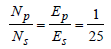∴ Es = 25 Ep
But EsIs = EpIp
25Ep × 2 = Ep × Ip ⇒ Ip = 5 0 A

31 Year NEET Previous Year Questions: Alternating Current - Question 12

An inductance L having a resistance R is connected to an alternating source of angular frequency ω. The quality factor Q of the
inductance is 

Detailed Solution for 31 Year NEET Previous Year Questions: Alternating Current - Question 12

Quality factor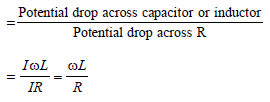31 Year NEET Previous Year Questions: Alternating Current - Question 13

A capacitor has capacity C and reactance X. Ifcapacitance and frequency become double, thenreactance will be 

Detailed Solution for 31 Year NEET Previous Year Questions: Alternating Current - Question 13

Capacitive reactance,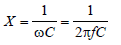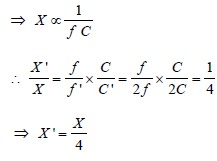31 Year NEET Previous Year Questions: Alternating Current - Question 14

In a series resonant circuit, having L, C and R as its elements, the resonant current is i. The power dissipated in the circuit at resonance is 

Detailed Solution for 31 Year NEET Previous Year Questions: Alternating Current - Question 14

At resonance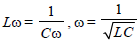Current through circuit i =E/R
Power dissipated at Resonance = i2R

31 Year NEET Previous Year Questions: Alternating Current - Question 15

A coil of 40 henry inductance is connected inseries with a resistance of 8 ohm and thecombination is joined to the terminals of a 2 voltbattery. The time constant of the circuit is    

Detailed Solution for 31 Year NEET Previous Year Questions: Alternating Current - Question 15

Time constant is L/R
Given, L = 40H & R = 8Ω
∴ τ = 40/8 = 5 sec

31 Year NEET Previous Year Questions: Alternating Current - Question 16

In a circuit, L, C and R are connected in series with an alternating voltage source of frequency f. The current leads the voltage by 45°. The value of C is 

Detailed Solution for 31 Year NEET Previous Year Questions: Alternating Current - Question 16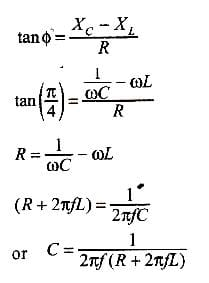31 Year NEET Previous Year Questions: Alternating Current - Question 17

A coil of inductive reactance 31 Ω has aresistance of 8 Ω. It is placed in series with acondenser of capacitative reactance 25Ω. Thecombination is connected to an a.c. source of110 volt. The power factor of the circuit is

Detailed Solution for 31 Year NEET Previous Year Questions: Alternating Current - Question 17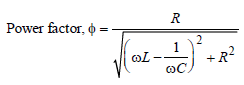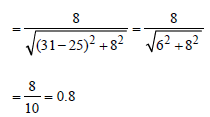31 Year NEET Previous Year Questions: Alternating Current - Question 18

The core of a transformer is laminated because        

Detailed Solution for 31 Year NEET Previous Year Questions: Alternating Current - Question 18

When there is change of flux in the core of a
transformer due to change in current around
it, eddy current is produced. The direction
of this current is opposite to the current
which produces it, so it will reduce the main
current. We laminate the core so that flux is
reduced resulting in the reduced production
of eddy current.

31 Year NEET Previous Year Questions: Alternating Current - Question 19

A transistor-oscillator using a resonant circuitwith an inductor L (of negligible resistance) anda capacitor C in series produce oscillations offrequency f. If L is doubled and C is changed to4C, the frequency will be 

Detailed Solution for 31 Year NEET Previous Year Questions: Alternating Current - Question 19

We know that frequency of electrical
oscillation in L.C. circuit is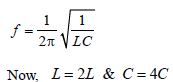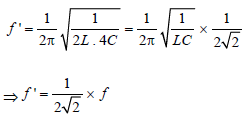31 Year NEET Previous Year Questions: Alternating Current - Question 20

The primary and secondary coil of a transformerhave 50 and 1500 turns respectively. If themagnetic flux φ linked with the primary coil isgiven by φ= φ0 + 4t, where φ is in webers, t is timein seconds and φ0 is a constant, the outputvoltage across the secondary coil is 

Detailed Solution for 31 Year NEET Previous Year Questions: Alternating Current - Question 20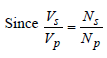Where
Ns = No. of turns across primary coil = 50
Np = No. of turns across secondary coil
= 1500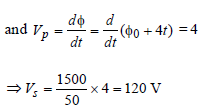31 Year NEET Previous Year Questions: Alternating Current - Question 21

A transformer is used to light a 100 W and 110 Vlamp from a 220 V mains. If the main current is 0.5amp, the efficiency of the transformer isapproximately 

Detailed Solution for 31 Year NEET Previous Year Questions: Alternating Current - Question 21

Efficiency of the transformer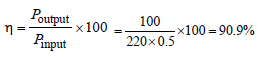31 Year NEET Previous Year Questions: Alternating Current - Question 22

What is the value of inductance L for which the current is maximum in a series LCR circuit with C = 10 μF and ω = 1000s -1 ? 

Detailed Solution for 31 Year NEET Previous Year Questions: Alternating Current - Question 22

Condition for which the current is maximum
in a series LCR circuit is,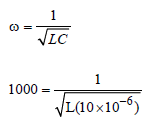= L = 100 mH

31 Year NEET Previous Year Questions: Alternating Current - Question 23

In an a.c circuit the e.m.f. (e) and the current (i) at any instant are given respectively by
e = E0 sin ωt         
i = I0 sin (ωt –φ)
The average power in the circuit over one cycle of a.c. is

Detailed Solution for 31 Year NEET Previous Year Questions: Alternating Current - Question 23

The average power in the circuit over one
cycle of a.c. is given by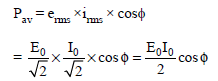31 Year NEET Previous Year Questions: Alternating Current - Question 24

Power dissipated in an LCR series circuit connected to an a.c source of emf εε is 

Detailed Solution for 31 Year NEET Previous Year Questions: Alternating Current - Question 24

Power dissipated in series LCR;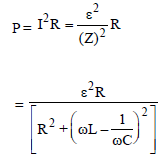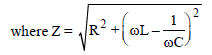is called the impedance of the circuit.

31 Year NEET Previous Year Questions: Alternating Current - Question 25

In the given circuit the reading of voltmeter V1 and V2 are 300 volts each. The reading of the voltmeter V3 and ammeter A are
respectively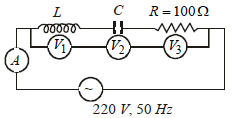Detailed Solution for 31 Year NEET Previous Year Questions: Alternating Current - Question 25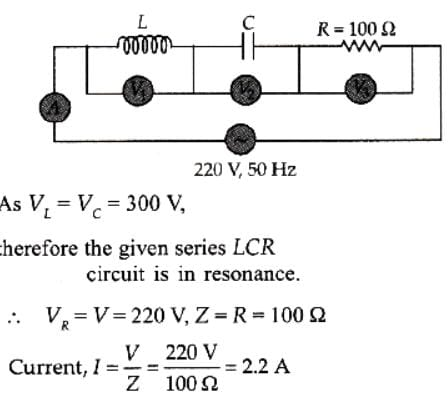Hence, the reading of the voltmeter V3 is 220 V and the reading of ammeter A is 2.2 A.

31 Year NEET Previous Year Questions: Alternating Current - Question 26

A 220 volts input is supplied to a transformer.The output circuit draws a current of 2.0 ampereat 440 volts. If the efficiency of the transformeris 80%, the current drawn by the primarywindings of the transformer is 

Detailed Solution for 31 Year NEET Previous Year Questions: Alternating Current - Question 26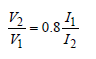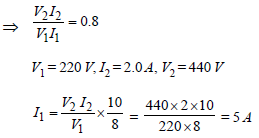31 Year NEET Previous Year Questions: Alternating Current - Question 27

An ac voltage is applied to a resistance R and aninductor L in series. If R and the inductivereactance are both equal to 3Ω, the phasedifference between the applied voltage and thecurrent in the circuit is 

Detailed Solution for 31 Year NEET Previous Year Questions: Alternating Current - Question 27

The phase difference φ is given by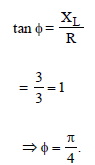31 Year NEET Previous Year Questions: Alternating Current - Question 28

In an ac circuit an alternating voltage e = 200 √2 sin 100 t volts is connected to a capacitorof capacity 1μF. The r.m.s. value of the currentin the circuit is 

Detailed Solution for 31 Year NEET Previous Year Questions: Alternating Current - Question 28

given e=200√2​sin100t
Erms​= 200√2​​/√2​
C=1×10−6
Z=Xc​=1/ωC​=1/100×10−6=104Ω
I=Erms​​/Z=(200√2​​​/√2​)/104 =2×10−2A=20mA

31 Year NEET Previous Year Questions: Alternating Current - Question 29

The r.m.s. value of potential difference V shown in the figure is [2011M]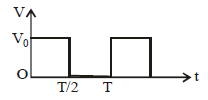Detailed Solution for 31 Year NEET Previous Year Questions: Alternating Current - Question 29

Voltage is given as    V=Vo​   for   0 ≥ t ≤ T/2
=0      for   T/2 ≥ t ≤T
Rms voltage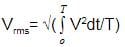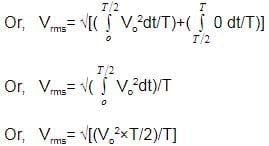⟹ Vrms​​=Vo​/√2​

31 Year NEET Previous Year Questions: Alternating Current - Question 30

A coil has resistance 30 ohm and inductive reactance 20 ohm at 50 Hz frequency. If an ac source, of 200 volt, 100 Hz, is connected across the coil, the current in the coil will be [2011M]

Detailed Solution for 31 Year NEET Previous Year Questions: Alternating Current - Question 30

If ω = 50 × 2π then ωL = 20Ω
If ω' = 100 × 2π then ω'L = 40Ω
Current flowing in the coil is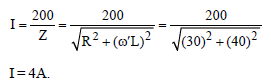31 Year NEET Previous Year Questions: Alternating Current - Question 31

In an electrical circuit R, L, C and an a.c. voltage source are all connected in series. When L is removed from the circuit, the phase difference between the voltage the current in the circuit is π/3. If instead, C is removed from the circuit, the phase difference is again π/3. The power factor of the circuit is : 

Detailed Solution for 31 Year NEET Previous Year Questions: Alternating Current - Question 31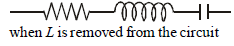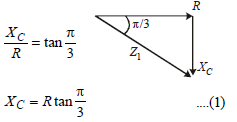when C is remove from the circuit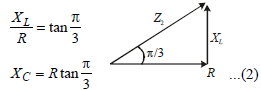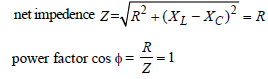31 Year NEET Previous Year Questions: Alternating Current - Question 32

The current (I) in the inductance is varying with time according to the plot shown in figure.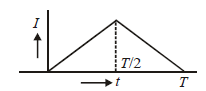Which one of the following is the correct variation of voltage with time in the coil?

Detailed Solution for 31 Year NEET Previous Year Questions: Alternating Current - Question 32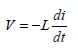Here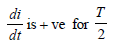time and di/dt  is -ve for next T/2 time so.

31 Year NEET Previous Year Questions: Alternating Current - Question 33

The instantaneous values of alternating current and voltages in a circuit are given as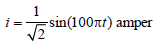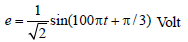The average power in Watts consumed in the circuit is : [2012M]

Detailed Solution for 31 Year NEET Previous Year Questions: Alternating Current - Question 33

The average power in the circuit where cos
φ = power factory

< P > = Vrms × Irms cos φ
φ = π/3 = phase difference =180/3 =  60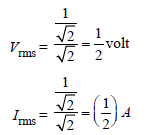31 Year NEET Previous Year Questions: Alternating Current - Question 34

A coil of self-inductance L is connected in serieswith a bulb B and an AC source. Brightness ofthe bulb decreases when

[NEET 2013]

Detailed Solution for 31 Year NEET Previous Year Questions: Alternating Current - Question 34

By inserting iron rod in the coil,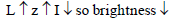31 Year NEET Previous Year Questions: Alternating Current - Question 35

The primary of a transformer when connected toa dc battery of 10 volt draws a current of 1 mA.The number of turns of the primary andsecondary windings are 50 and 100 respectively.The voltage in the secondary and the currentdrawn by the circuit in the secondary arerespectively [NEET Kar. 2013]

Detailed Solution for 31 Year NEET Previous Year Questions: Alternating Current - Question 35

A transformer is essentially an AC device.
DC source so no mutual induction between
coils
⇒ E2 = 0 and I2 = 0

## Physics Class 12

157 videos|452 docs|213 tests
 Use Code STAYHOME200 and get INR 200 additional OFF Use Coupon Code
Information about 31 Year NEET Previous Year Questions: Alternating Current Page
In this test you can find the Exam questions for 31 Year NEET Previous Year Questions: Alternating Current solved & explained in the simplest way possible. Besides giving Questions and answers for 31 Year NEET Previous Year Questions: Alternating Current, EduRev gives you an ample number of Online tests for practice

## Physics Class 12

157 videos|452 docs|213 tests

### How to Prepare for NEET

Read our guide to prepare for NEET which is created by Toppers & the best Teachers